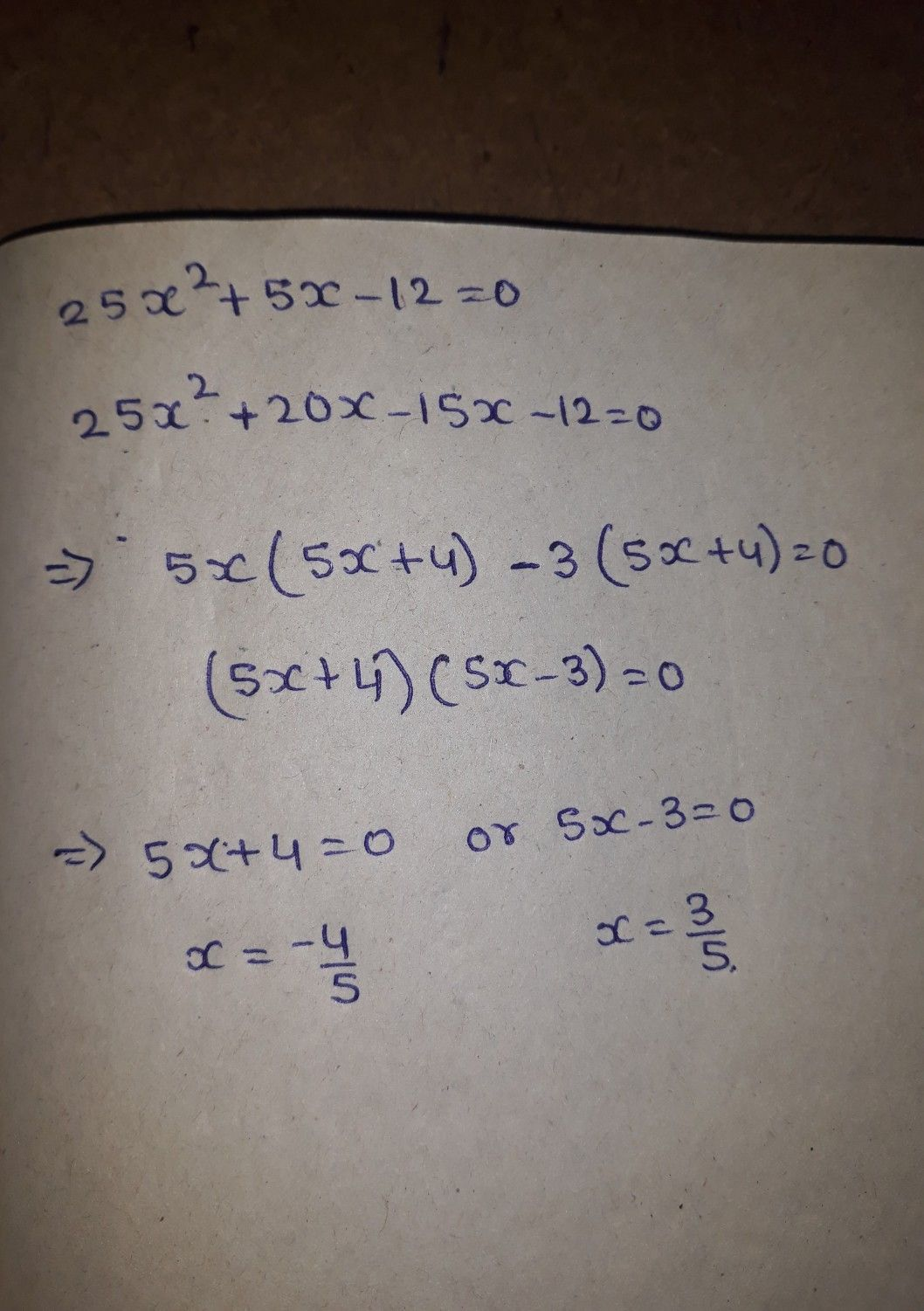Symbol
ProblemSolve by $taclo\pi mg$ $2sx^{2}+5x-12-0$ Please enter any square root values using "sqrt" or the decimal answer rounded to the nearest hundredth $\left(1.c.\sqrt{2} =$ $sa\pi \left(2$ $1\left(2\right)0t$ $1.41\right)$